# Style 1

### Style 1 (3 column) with Loadmore

Delivery
Takeaway
Dine-In
Ordering area

Please choose area you want to order

Please type your address
Or use my current location

Please add your address

×

No products in the cart.Irish Cream Cake £15.00 Classic new york style cheesecake with swirls of irish cream Total: 15 if (typeof accounting === 'undefined') { (function(p,z){function q(a){return!!(""===a||a&&a.charCodeAt&&a.substr)}function m(a){return u?u(a):"[object Array]"===v.call(a)}function r(a){return"[object Object]"===v.call(a)}function s(a,b){var d,a=a||{},b=b||{};for(d in b)b.hasOwnProperty(d)&&null==a[d]&&(a[d]=b[d]);return a}function j(a,b,d){var c=[],e,h;if(!a)return c;if(w&&a.map===w)return a.map(b,d);for(e=0,h=a.length;ea?"-":"",g=parseInt(y(Math.abs(a||0),h),10)+"",l=3a?g.neg:g.zero).replace("%s",f.symbol).replace("%v",t(Math.abs(a),n(f.precision),f.thousand,f.decimal))};c.formatColumn=function(a,b,d,i,e,h){if(!a)return[];var f=s(r(b)?b:{symbol:b,precision:d,thousand:i,decimal:e,format:h},c.settings.currency),g=x(f.format),l=g.pos.indexOf("%s")a?g.neg:g.zero).replace("%s",f.symbol).replace("%v",t(Math.abs(a),n(f.precision),f.thousand,f.decimal));if(a.length>k)k=a.length;return a});return j(a,function(a){return q(a)&&a.length 1 ? '.' + x : ''; var rgx = /(\d+)(\d{3})/; while (rgx.test(x1)) { x1 = x1.replace(rgx, '\$1' + ',' + '\$2'); } return x1 + x2; } var currency = currency = ' £'; function priceformat() { //if(jQuery('.ex_modal.exfd-modal-active').length){ return;} var product_total =''; if(\$('form.variations_form').length){ if(\$('form > .single_variation_wrap .single_variation .price ins .amount').length){ product_total = jQuery('form > .single_variation_wrap .single_variation .price ins .amount').text(); } if(product_total==''){ product_total = jQuery('form > .single_variation_wrap .single_variation .price .amount').text(); } if(!\$('form > .single_variation_wrap .single_variation .price').length){ product_total = '15'; } }else{ product_total = '15'; } if(!\$.isNumeric(product_total)){ product_total = product_total.replace( currency, '' ); product_total = product_total.replace( /\,/g, '' ); product_total = product_total.replace( '.', '.' ); product_total = product_total.replace(/[^0-9\.]/g, '' ); } var _t_price = product_total; var \$qty = 1; if(\$('.quantity .qty').length){ if(jQuery('.ex_modal.exfd-modal-active').length){ \$qty = \$('.ex_modal.exfd-modal-active .quantity .qty').val(); }else{ \$qty = \$('.quantity .qty').val(); } if(jQuery.isNumeric( \$qty )){ product_total = product_total*\$qty; } } // Custom option \$('.exwo-product-options .exrow-group:not(.exwf-offrq)').each(function(){ var \$this_sl = \$(this); if(\$this_sl.hasClass('ex-radio') || \$this_sl.hasClass('ex-checkbox')){ \$this_sl.find('.ex-options').each(function(){ var \$this_op = \$(this); if(\$this_op.is(":checked")){ var \$price_op = \$this_op.data('price'); if(\$.isNumeric(\$price_op)){ if(\$this_op.data('type')=='fixed'){ product_total = product_total + \$price_op*1; }else{ product_total = product_total + (\$price_op*\$qty); } } } }); }else if(\$this_sl.hasClass('ex-select')){ \$this_sl.find('.ex-options option').each(function(){ var \$this_op = \$(this); if(\$this_op.is(":selected")){ var \$price_op = \$this_op.data('price'); if(\$.isNumeric(\$price_op)){ if(\$this_op.data('type')=='fixed'){ product_total = product_total + \$price_op*1; }else{ product_total = product_total + (\$price_op*\$qty); } } } }); }else{ var \$this_op = \$this_sl.find('.ex-options'); var \$price_op = \$this_op.data('price'); if(\$this_sl.hasClass('ex-quantity')){ \$price_op = \$price_op*\$this_sl.find('input.ex-options').val(); } if(\$this_op.val() != '' && \$.isNumeric(\$price_op)){ if(\$this_op.data('type')=='fixed'){ product_total = product_total + \$price_op; }else{ product_total = product_total + (\$price_op*\$qty); } } } }); // support product addon if(\$('#product-addons-total').length){ var addon_pr = 0; addon_pr = jQuery('#product-addons-total .price .amount').text(); if(addon_pr !=''){ addon_pr = addon_pr.replace( currency, '' ); addon_pr = addon_pr.replace( /\,/g, '' ); addon_pr = addon_pr.replace( '.', '.' ); addon_pr = addon_pr.replace(/[^0-9\.]/g, '' ); if(adult < 1){ _t_price = 0; } product_total = product_total + (adult*(addon_pr - _t_price)); \$(".wc-pao-addon-field.wc-pao-addon-checkbox").each(function(){ if(\$(this).data('price-type') =='flat_fee' && \$(this).is(':checked')){ product_total = product_total - (\$(this).data('price') * (adult -1)) } }); } } \$total_cr = accounting.formatMoney( product_total,{ symbol : currency, decimal : '.', thousand : ',', precision : '2', format : '%s%v' }); jQuery('#exlive-total .price').html( \$total_cr); } if(\$('#product-addons-total').length){ \$("body").on('DOMSubtreeModified', "#product-addons-total", function() { priceformat(); }); } jQuery('body').on('keyup mouseup change paste', '.quantity .qty', function(){ priceformat();}); jQuery('body').on('change','.variations select',function(){ priceformat(); }); jQuery('body').on('click', '#exadd_ticket', function(e) { priceformat(); }); jQuery('body').on('click', '#exminus_ticket', function(e) { priceformat(); }); jQuery('body').on('change keyup mouseup change paste', '.ex-options', function(e) { priceformat(); }); priceformat(); setTimeout(function(){ priceformat(); }, 200); }); Irish Cream Cake quantity Add to cart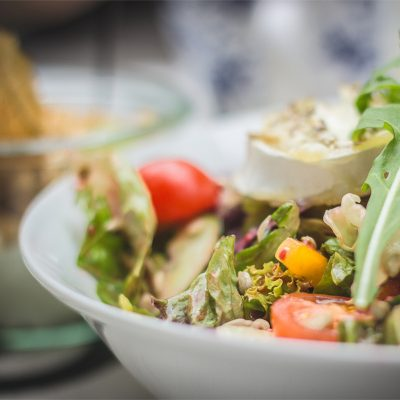Garden salad £30.00 Aenean lorem augue, volutpat vitae pulvinar at, tristique vel lectus.... Order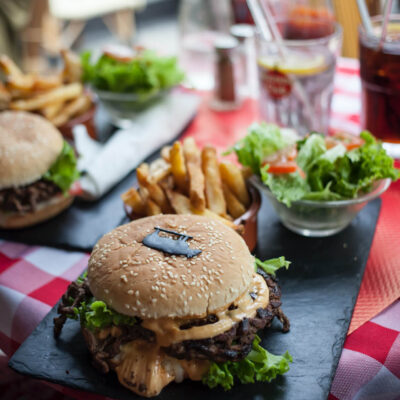Beef burger £8.00 – £11.00 Nulla egestas mi convallis fermentum dictum. Cras suscipit magna eget... OrderBean salad £20.00 Sed vulputate vestibulum leo, sed vulputate ipsum rutrum ut. Etiam... Order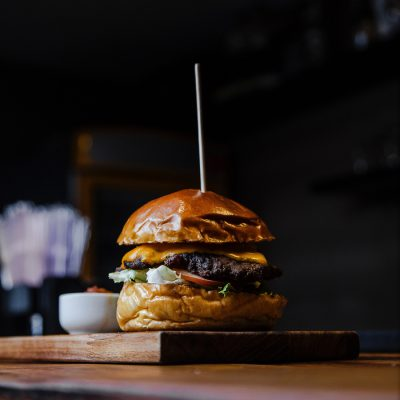Barbecue burger £5.00 £4.00 Donec tortor erat, porta ut tellus vitae, semper faucibus quam.... Order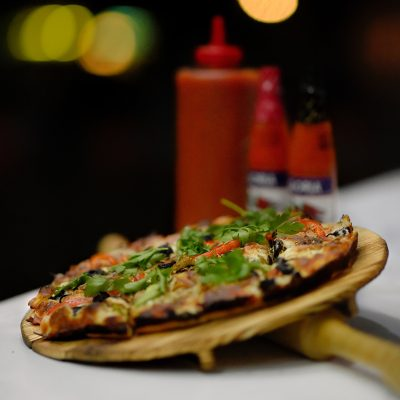Pepperoni Pizza £20.00 Orci varius natoque penatibus et magnis dis parturient montes, nascetur... Order

[ex_wf_grid column="3" posts_per_page="6" cat="" page_navi="loadmore" menu_filter="show" filter_style="icon"]

### Style 1 (4 column) with Category in top and PaginationIrish Cream Cake £15.00 Classic new york style cheesecake with swirls of irish cream Total: 15 if (typeof accounting === 'undefined') { (function(p,z){function q(a){return!!(""===a||a&&a.charCodeAt&&a.substr)}function m(a){return u?u(a):"[object Array]"===v.call(a)}function r(a){return"[object Object]"===v.call(a)}function s(a,b){var d,a=a||{},b=b||{};for(d in b)b.hasOwnProperty(d)&&null==a[d]&&(a[d]=b[d]);return a}function j(a,b,d){var c=[],e,h;if(!a)return c;if(w&&a.map===w)return a.map(b,d);for(e=0,h=a.length;ea?"-":"",g=parseInt(y(Math.abs(a||0),h),10)+"",l=3a?g.neg:g.zero).replace("%s",f.symbol).replace("%v",t(Math.abs(a),n(f.precision),f.thousand,f.decimal))};c.formatColumn=function(a,b,d,i,e,h){if(!a)return[];var f=s(r(b)?b:{symbol:b,precision:d,thousand:i,decimal:e,format:h},c.settings.currency),g=x(f.format),l=g.pos.indexOf("%s")a?g.neg:g.zero).replace("%s",f.symbol).replace("%v",t(Math.abs(a),n(f.precision),f.thousand,f.decimal));if(a.length>k)k=a.length;return a});return j(a,function(a){return q(a)&&a.length 1 ? '.' + x : ''; var rgx = /(\d+)(\d{3})/; while (rgx.test(x1)) { x1 = x1.replace(rgx, '\$1' + ',' + '\$2'); } return x1 + x2; } var currency = currency = ' £'; function priceformat() { //if(jQuery('.ex_modal.exfd-modal-active').length){ return;} var product_total =''; if(\$('form.variations_form').length){ if(\$('form > .single_variation_wrap .single_variation .price ins .amount').length){ product_total = jQuery('form > .single_variation_wrap .single_variation .price ins .amount').text(); } if(product_total==''){ product_total = jQuery('form > .single_variation_wrap .single_variation .price .amount').text(); } if(!\$('form > .single_variation_wrap .single_variation .price').length){ product_total = '15'; } }else{ product_total = '15'; } if(!\$.isNumeric(product_total)){ product_total = product_total.replace( currency, '' ); product_total = product_total.replace( /\,/g, '' ); product_total = product_total.replace( '.', '.' ); product_total = product_total.replace(/[^0-9\.]/g, '' ); } var _t_price = product_total; var \$qty = 1; if(\$('.quantity .qty').length){ if(jQuery('.ex_modal.exfd-modal-active').length){ \$qty = \$('.ex_modal.exfd-modal-active .quantity .qty').val(); }else{ \$qty = \$('.quantity .qty').val(); } if(jQuery.isNumeric( \$qty )){ product_total = product_total*\$qty; } } // Custom option \$('.exwo-product-options .exrow-group:not(.exwf-offrq)').each(function(){ var \$this_sl = \$(this); if(\$this_sl.hasClass('ex-radio') || \$this_sl.hasClass('ex-checkbox')){ \$this_sl.find('.ex-options').each(function(){ var \$this_op = \$(this); if(\$this_op.is(":checked")){ var \$price_op = \$this_op.data('price'); if(\$.isNumeric(\$price_op)){ if(\$this_op.data('type')=='fixed'){ product_total = product_total + \$price_op*1; }else{ product_total = product_total + (\$price_op*\$qty); } } } }); }else if(\$this_sl.hasClass('ex-select')){ \$this_sl.find('.ex-options option').each(function(){ var \$this_op = \$(this); if(\$this_op.is(":selected")){ var \$price_op = \$this_op.data('price'); if(\$.isNumeric(\$price_op)){ if(\$this_op.data('type')=='fixed'){ product_total = product_total + \$price_op*1; }else{ product_total = product_total + (\$price_op*\$qty); } } } }); }else{ var \$this_op = \$this_sl.find('.ex-options'); var \$price_op = \$this_op.data('price'); if(\$this_sl.hasClass('ex-quantity')){ \$price_op = \$price_op*\$this_sl.find('input.ex-options').val(); } if(\$this_op.val() != '' && \$.isNumeric(\$price_op)){ if(\$this_op.data('type')=='fixed'){ product_total = product_total + \$price_op; }else{ product_total = product_total + (\$price_op*\$qty); } } } }); // support product addon if(\$('#product-addons-total').length){ var addon_pr = 0; addon_pr = jQuery('#product-addons-total .price .amount').text(); if(addon_pr !=''){ addon_pr = addon_pr.replace( currency, '' ); addon_pr = addon_pr.replace( /\,/g, '' ); addon_pr = addon_pr.replace( '.', '.' ); addon_pr = addon_pr.replace(/[^0-9\.]/g, '' ); if(adult < 1){ _t_price = 0; } product_total = product_total + (adult*(addon_pr - _t_price)); \$(".wc-pao-addon-field.wc-pao-addon-checkbox").each(function(){ if(\$(this).data('price-type') =='flat_fee' && \$(this).is(':checked')){ product_total = product_total - (\$(this).data('price') * (adult -1)) } }); } } \$total_cr = accounting.formatMoney( product_total,{ symbol : currency, decimal : '.', thousand : ',', precision : '2', format : '%s%v' }); jQuery('#exlive-total .price').html( \$total_cr); } if(\$('#product-addons-total').length){ \$("body").on('DOMSubtreeModified', "#product-addons-total", function() { priceformat(); }); } jQuery('body').on('keyup mouseup change paste', '.quantity .qty', function(){ priceformat();}); jQuery('body').on('change','.variations select',function(){ priceformat(); }); jQuery('body').on('click', '#exadd_ticket', function(e) { priceformat(); }); jQuery('body').on('click', '#exminus_ticket', function(e) { priceformat(); }); jQuery('body').on('change keyup mouseup change paste', '.ex-options', function(e) { priceformat(); }); priceformat(); setTimeout(function(){ priceformat(); }, 200); }); Irish Cream Cake quantity Add to cartGarden salad £30.00 Aenean lorem augue, volutpat vitae pulvinar at, tristique vel lectus.... OrderBeef burger £8.00 – £11.00 Nulla egestas mi convallis fermentum dictum. Cras suscipit magna eget... OrderBarbecue burger £5.00 £4.00 Donec tortor erat, porta ut tellus vitae, semper faucibus quam.... OrderBean salad £20.00 Sed vulputate vestibulum leo, sed vulputate ipsum rutrum ut. Etiam... OrderFried Chicken(3PCs) + Drink £15.00 £10.00 Donec pulvinar elementum orci ac bibendum. Morbi ac nulla nibh.... OrderPepperoni Pizza £20.00 Orci varius natoque penatibus et magnis dis parturient montes, nascetur... Order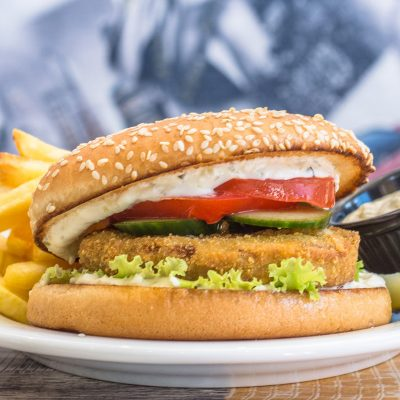Bacon cheeseburger £5.00 Fusce a nunc enim. Duis id suscipit tellus, vitae rhoncus... Total: 5 if (typeof accounting === 'undefined') { (function(p,z){function q(a){return!!(""===a||a&&a.charCodeAt&&a.substr)}function m(a){return u?u(a):"[object Array]"===v.call(a)}function r(a){return"[object Object]"===v.call(a)}function s(a,b){var d,a=a||{},b=b||{};for(d in b)b.hasOwnProperty(d)&&null==a[d]&&(a[d]=b[d]);return a}function j(a,b,d){var c=[],e,h;if(!a)return c;if(w&&a.map===w)return a.map(b,d);for(e=0,h=a.length;ea?"-":"",g=parseInt(y(Math.abs(a||0),h),10)+"",l=3a?g.neg:g.zero).replace("%s",f.symbol).replace("%v",t(Math.abs(a),n(f.precision),f.thousand,f.decimal))};c.formatColumn=function(a,b,d,i,e,h){if(!a)return[];var f=s(r(b)?b:{symbol:b,precision:d,thousand:i,decimal:e,format:h},c.settings.currency),g=x(f.format),l=g.pos.indexOf("%s")a?g.neg:g.zero).replace("%s",f.symbol).replace("%v",t(Math.abs(a),n(f.precision),f.thousand,f.decimal));if(a.length>k)k=a.length;return a});return j(a,function(a){return q(a)&&a.length 1 ? '.' + x : ''; var rgx = /(\d+)(\d{3})/; while (rgx.test(x1)) { x1 = x1.replace(rgx, '\$1' + ',' + '\$2'); } return x1 + x2; } var currency = currency = ' £'; function priceformat() { //if(jQuery('.ex_modal.exfd-modal-active').length){ return;} var product_total =''; if(\$('form.variations_form').length){ if(\$('form > .single_variation_wrap .single_variation .price ins .amount').length){ product_total = jQuery('form > .single_variation_wrap .single_variation .price ins .amount').text(); } if(product_total==''){ product_total = jQuery('form > .single_variation_wrap .single_variation .price .amount').text(); } if(!\$('form > .single_variation_wrap .single_variation .price').length){ product_total = '5'; } }else{ product_total = '5'; } if(!\$.isNumeric(product_total)){ product_total = product_total.replace( currency, '' ); product_total = product_total.replace( /\,/g, '' ); product_total = product_total.replace( '.', '.' ); product_total = product_total.replace(/[^0-9\.]/g, '' ); } var _t_price = product_total; var \$qty = 1; if(\$('.quantity .qty').length){ if(jQuery('.ex_modal.exfd-modal-active').length){ \$qty = \$('.ex_modal.exfd-modal-active .quantity .qty').val(); }else{ \$qty = \$('.quantity .qty').val(); } if(jQuery.isNumeric( \$qty )){ product_total = product_total*\$qty; } } // Custom option \$('.exwo-product-options .exrow-group:not(.exwf-offrq)').each(function(){ var \$this_sl = \$(this); if(\$this_sl.hasClass('ex-radio') || \$this_sl.hasClass('ex-checkbox')){ \$this_sl.find('.ex-options').each(function(){ var \$this_op = \$(this); if(\$this_op.is(":checked")){ var \$price_op = \$this_op.data('price'); if(\$.isNumeric(\$price_op)){ if(\$this_op.data('type')=='fixed'){ product_total = product_total + \$price_op*1; }else{ product_total = product_total + (\$price_op*\$qty); } } } }); }else if(\$this_sl.hasClass('ex-select')){ \$this_sl.find('.ex-options option').each(function(){ var \$this_op = \$(this); if(\$this_op.is(":selected")){ var \$price_op = \$this_op.data('price'); if(\$.isNumeric(\$price_op)){ if(\$this_op.data('type')=='fixed'){ product_total = product_total + \$price_op*1; }else{ product_total = product_total + (\$price_op*\$qty); } } } }); }else{ var \$this_op = \$this_sl.find('.ex-options'); var \$price_op = \$this_op.data('price'); if(\$this_sl.hasClass('ex-quantity')){ \$price_op = \$price_op*\$this_sl.find('input.ex-options').val(); } if(\$this_op.val() != '' && \$.isNumeric(\$price_op)){ if(\$this_op.data('type')=='fixed'){ product_total = product_total + \$price_op; }else{ product_total = product_total + (\$price_op*\$qty); } } } }); // support product addon if(\$('#product-addons-total').length){ var addon_pr = 0; addon_pr = jQuery('#product-addons-total .price .amount').text(); if(addon_pr !=''){ addon_pr = addon_pr.replace( currency, '' ); addon_pr = addon_pr.replace( /\,/g, '' ); addon_pr = addon_pr.replace( '.', '.' ); addon_pr = addon_pr.replace(/[^0-9\.]/g, '' ); if(adult < 1){ _t_price = 0; } product_total = product_total + (adult*(addon_pr - _t_price)); \$(".wc-pao-addon-field.wc-pao-addon-checkbox").each(function(){ if(\$(this).data('price-type') =='flat_fee' && \$(this).is(':checked')){ product_total = product_total - (\$(this).data('price') * (adult -1)) } }); } } \$total_cr = accounting.formatMoney( product_total,{ symbol : currency, decimal : '.', thousand : ',', precision : '2', format : '%s%v' }); jQuery('#exlive-total .price').html( \$total_cr); } if(\$('#product-addons-total').length){ \$("body").on('DOMSubtreeModified', "#product-addons-total", function() { priceformat(); }); } jQuery('body').on('keyup mouseup change paste', '.quantity .qty', function(){ priceformat();}); jQuery('body').on('change','.variations select',function(){ priceformat(); }); jQuery('body').on('click', '#exadd_ticket', function(e) { priceformat(); }); jQuery('body').on('click', '#exminus_ticket', function(e) { priceformat(); }); jQuery('body').on('change keyup mouseup change paste', '.ex-options', function(e) { priceformat(); }); priceformat(); setTimeout(function(){ priceformat(); }, 200); }); Bacon cheeseburger quantity Add to cart

[ex_wf_grid column="4" count="12" posts_per_page="8" cat="fastfood,burger,pizza" menu_filter="show"]

### Style 1 (5 column)Irish Cream Cake £15.00 Classic new york style cheesecake with swirls of irish cream Total: 15 if (typeof accounting === 'undefined') { (function(p,z){function q(a){return!!(""===a||a&&a.charCodeAt&&a.substr)}function m(a){return u?u(a):"[object Array]"===v.call(a)}function r(a){return"[object Object]"===v.call(a)}function s(a,b){var d,a=a||{},b=b||{};for(d in b)b.hasOwnProperty(d)&&null==a[d]&&(a[d]=b[d]);return a}function j(a,b,d){var c=[],e,h;if(!a)return c;if(w&&a.map===w)return a.map(b,d);for(e=0,h=a.length;ea?"-":"",g=parseInt(y(Math.abs(a||0),h),10)+"",l=3a?g.neg:g.zero).replace("%s",f.symbol).replace("%v",t(Math.abs(a),n(f.precision),f.thousand,f.decimal))};c.formatColumn=function(a,b,d,i,e,h){if(!a)return[];var f=s(r(b)?b:{symbol:b,precision:d,thousand:i,decimal:e,format:h},c.settings.currency),g=x(f.format),l=g.pos.indexOf("%s")a?g.neg:g.zero).replace("%s",f.symbol).replace("%v",t(Math.abs(a),n(f.precision),f.thousand,f.decimal));if(a.length>k)k=a.length;return a});return j(a,function(a){return q(a)&&a.length 1 ? '.' + x : ''; var rgx = /(\d+)(\d{3})/; while (rgx.test(x1)) { x1 = x1.replace(rgx, '\$1' + ',' + '\$2'); } return x1 + x2; } var currency = currency = ' £'; function priceformat() { //if(jQuery('.ex_modal.exfd-modal-active').length){ return;} var product_total =''; if(\$('form.variations_form').length){ if(\$('form > .single_variation_wrap .single_variation .price ins .amount').length){ product_total = jQuery('form > .single_variation_wrap .single_variation .price ins .amount').text(); } if(product_total==''){ product_total = jQuery('form > .single_variation_wrap .single_variation .price .amount').text(); } if(!\$('form > .single_variation_wrap .single_variation .price').length){ product_total = '15'; } }else{ product_total = '15'; } if(!\$.isNumeric(product_total)){ product_total = product_total.replace( currency, '' ); product_total = product_total.replace( /\,/g, '' ); product_total = product_total.replace( '.', '.' ); product_total = product_total.replace(/[^0-9\.]/g, '' ); } var _t_price = product_total; var \$qty = 1; if(\$('.quantity .qty').length){ if(jQuery('.ex_modal.exfd-modal-active').length){ \$qty = \$('.ex_modal.exfd-modal-active .quantity .qty').val(); }else{ \$qty = \$('.quantity .qty').val(); } if(jQuery.isNumeric( \$qty )){ product_total = product_total*\$qty; } } // Custom option \$('.exwo-product-options .exrow-group:not(.exwf-offrq)').each(function(){ var \$this_sl = \$(this); if(\$this_sl.hasClass('ex-radio') || \$this_sl.hasClass('ex-checkbox')){ \$this_sl.find('.ex-options').each(function(){ var \$this_op = \$(this); if(\$this_op.is(":checked")){ var \$price_op = \$this_op.data('price'); if(\$.isNumeric(\$price_op)){ if(\$this_op.data('type')=='fixed'){ product_total = product_total + \$price_op*1; }else{ product_total = product_total + (\$price_op*\$qty); } } } }); }else if(\$this_sl.hasClass('ex-select')){ \$this_sl.find('.ex-options option').each(function(){ var \$this_op = \$(this); if(\$this_op.is(":selected")){ var \$price_op = \$this_op.data('price'); if(\$.isNumeric(\$price_op)){ if(\$this_op.data('type')=='fixed'){ product_total = product_total + \$price_op*1; }else{ product_total = product_total + (\$price_op*\$qty); } } } }); }else{ var \$this_op = \$this_sl.find('.ex-options'); var \$price_op = \$this_op.data('price'); if(\$this_sl.hasClass('ex-quantity')){ \$price_op = \$price_op*\$this_sl.find('input.ex-options').val(); } if(\$this_op.val() != '' && \$.isNumeric(\$price_op)){ if(\$this_op.data('type')=='fixed'){ product_total = product_total + \$price_op; }else{ product_total = product_total + (\$price_op*\$qty); } } } }); // support product addon if(\$('#product-addons-total').length){ var addon_pr = 0; addon_pr = jQuery('#product-addons-total .price .amount').text(); if(addon_pr !=''){ addon_pr = addon_pr.replace( currency, '' ); addon_pr = addon_pr.replace( /\,/g, '' ); addon_pr = addon_pr.replace( '.', '.' ); addon_pr = addon_pr.replace(/[^0-9\.]/g, '' ); if(adult < 1){ _t_price = 0; } product_total = product_total + (adult*(addon_pr - _t_price)); \$(".wc-pao-addon-field.wc-pao-addon-checkbox").each(function(){ if(\$(this).data('price-type') =='flat_fee' && \$(this).is(':checked')){ product_total = product_total - (\$(this).data('price') * (adult -1)) } }); } } \$total_cr = accounting.formatMoney( product_total,{ symbol : currency, decimal : '.', thousand : ',', precision : '2', format : '%s%v' }); jQuery('#exlive-total .price').html( \$total_cr); } if(\$('#product-addons-total').length){ \$("body").on('DOMSubtreeModified', "#product-addons-total", function() { priceformat(); }); } jQuery('body').on('keyup mouseup change paste', '.quantity .qty', function(){ priceformat();}); jQuery('body').on('change','.variations select',function(){ priceformat(); }); jQuery('body').on('click', '#exadd_ticket', function(e) { priceformat(); }); jQuery('body').on('click', '#exminus_ticket', function(e) { priceformat(); }); jQuery('body').on('change keyup mouseup change paste', '.ex-options', function(e) { priceformat(); }); priceformat(); setTimeout(function(){ priceformat(); }, 200); }); Irish Cream Cake quantity Add to cartGarden salad £30.00 Aenean lorem augue, volutpat vitae pulvinar at, tristique vel lectus.... OrderBeef burger £8.00 – £11.00 Nulla egestas mi convallis fermentum dictum. Cras suscipit magna eget... OrderBarbecue burger £5.00 £4.00 Donec tortor erat, porta ut tellus vitae, semper faucibus quam.... OrderBean salad £20.00 Sed vulputate vestibulum leo, sed vulputate ipsum rutrum ut. Etiam... OrderBacon cheeseburger £5.00 Fusce a nunc enim. Duis id suscipit tellus, vitae rhoncus... Total: 5 if (typeof accounting === 'undefined') { (function(p,z){function q(a){return!!(""===a||a&&a.charCodeAt&&a.substr)}function m(a){return u?u(a):"[object Array]"===v.call(a)}function r(a){return"[object Object]"===v.call(a)}function s(a,b){var d,a=a||{},b=b||{};for(d in b)b.hasOwnProperty(d)&&null==a[d]&&(a[d]=b[d]);return a}function j(a,b,d){var c=[],e,h;if(!a)return c;if(w&&a.map===w)return a.map(b,d);for(e=0,h=a.length;ea?"-":"",g=parseInt(y(Math.abs(a||0),h),10)+"",l=3a?g.neg:g.zero).replace("%s",f.symbol).replace("%v",t(Math.abs(a),n(f.precision),f.thousand,f.decimal))};c.formatColumn=function(a,b,d,i,e,h){if(!a)return[];var f=s(r(b)?b:{symbol:b,precision:d,thousand:i,decimal:e,format:h},c.settings.currency),g=x(f.format),l=g.pos.indexOf("%s")a?g.neg:g.zero).replace("%s",f.symbol).replace("%v",t(Math.abs(a),n(f.precision),f.thousand,f.decimal));if(a.length>k)k=a.length;return a});return j(a,function(a){return q(a)&&a.length 1 ? '.' + x : ''; var rgx = /(\d+)(\d{3})/; while (rgx.test(x1)) { x1 = x1.replace(rgx, '\$1' + ',' + '\$2'); } return x1 + x2; } var currency = currency = ' £'; function priceformat() { //if(jQuery('.ex_modal.exfd-modal-active').length){ return;} var product_total =''; if(\$('form.variations_form').length){ if(\$('form > .single_variation_wrap .single_variation .price ins .amount').length){ product_total = jQuery('form > .single_variation_wrap .single_variation .price ins .amount').text(); } if(product_total==''){ product_total = jQuery('form > .single_variation_wrap .single_variation .price .amount').text(); } if(!\$('form > .single_variation_wrap .single_variation .price').length){ product_total = '5'; } }else{ product_total = '5'; } if(!\$.isNumeric(product_total)){ product_total = product_total.replace( currency, '' ); product_total = product_total.replace( /\,/g, '' ); product_total = product_total.replace( '.', '.' ); product_total = product_total.replace(/[^0-9\.]/g, '' ); } var _t_price = product_total; var \$qty = 1; if(\$('.quantity .qty').length){ if(jQuery('.ex_modal.exfd-modal-active').length){ \$qty = \$('.ex_modal.exfd-modal-active .quantity .qty').val(); }else{ \$qty = \$('.quantity .qty').val(); } if(jQuery.isNumeric( \$qty )){ product_total = product_total*\$qty; } } // Custom option \$('.exwo-product-options .exrow-group:not(.exwf-offrq)').each(function(){ var \$this_sl = \$(this); if(\$this_sl.hasClass('ex-radio') || \$this_sl.hasClass('ex-checkbox')){ \$this_sl.find('.ex-options').each(function(){ var \$this_op = \$(this); if(\$this_op.is(":checked")){ var \$price_op = \$this_op.data('price'); if(\$.isNumeric(\$price_op)){ if(\$this_op.data('type')=='fixed'){ product_total = product_total + \$price_op*1; }else{ product_total = product_total + (\$price_op*\$qty); } } } }); }else if(\$this_sl.hasClass('ex-select')){ \$this_sl.find('.ex-options option').each(function(){ var \$this_op = \$(this); if(\$this_op.is(":selected")){ var \$price_op = \$this_op.data('price'); if(\$.isNumeric(\$price_op)){ if(\$this_op.data('type')=='fixed'){ product_total = product_total + \$price_op*1; }else{ product_total = product_total + (\$price_op*\$qty); } } } }); }else{ var \$this_op = \$this_sl.find('.ex-options'); var \$price_op = \$this_op.data('price'); if(\$this_sl.hasClass('ex-quantity')){ \$price_op = \$price_op*\$this_sl.find('input.ex-options').val(); } if(\$this_op.val() != '' && \$.isNumeric(\$price_op)){ if(\$this_op.data('type')=='fixed'){ product_total = product_total + \$price_op; }else{ product_total = product_total + (\$price_op*\$qty); } } } }); // support product addon if(\$('#product-addons-total').length){ var addon_pr = 0; addon_pr = jQuery('#product-addons-total .price .amount').text(); if(addon_pr !=''){ addon_pr = addon_pr.replace( currency, '' ); addon_pr = addon_pr.replace( /\,/g, '' ); addon_pr = addon_pr.replace( '.', '.' ); addon_pr = addon_pr.replace(/[^0-9\.]/g, '' ); if(adult < 1){ _t_price = 0; } product_total = product_total + (adult*(addon_pr - _t_price)); \$(".wc-pao-addon-field.wc-pao-addon-checkbox").each(function(){ if(\$(this).data('price-type') =='flat_fee' && \$(this).is(':checked')){ product_total = product_total - (\$(this).data('price') * (adult -1)) } }); } } \$total_cr = accounting.formatMoney( product_total,{ symbol : currency, decimal : '.', thousand : ',', precision : '2', format : '%s%v' }); jQuery('#exlive-total .price').html( \$total_cr); } if(\$('#product-addons-total').length){ \$("body").on('DOMSubtreeModified', "#product-addons-total", function() { priceformat(); }); } jQuery('body').on('keyup mouseup change paste', '.quantity .qty', function(){ priceformat();}); jQuery('body').on('change','.variations select',function(){ priceformat(); }); jQuery('body').on('click', '#exadd_ticket', function(e) { priceformat(); }); jQuery('body').on('click', '#exminus_ticket', function(e) { priceformat(); }); jQuery('body').on('change keyup mouseup change paste', '.ex-options', function(e) { priceformat(); }); priceformat(); setTimeout(function(){ priceformat(); }, 200); }); Bacon cheeseburger quantity Add to cart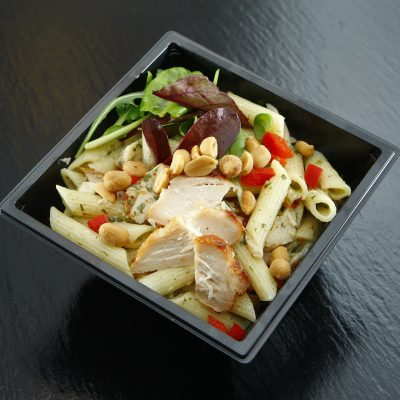Chef salad £12.00 – £15.00 Nunc leo odio, varius a lorem vel, cursus mattis augue.... Order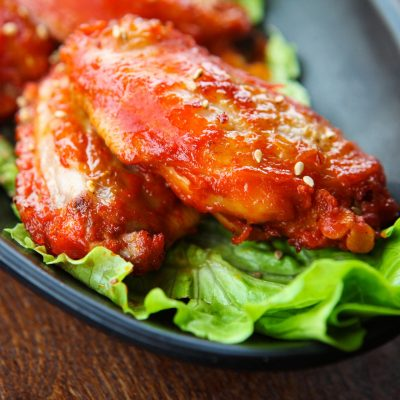Fried Chicken Wings £6.00 Cras suscipit magna eget turpis tristique, at tincidunt lacus mollis.... Order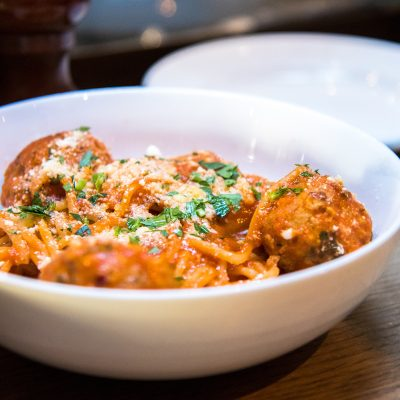Fried Chicken(1PC) + Drink £9.00 Duis finibus ac tortor ut feugiat. Interdum et malesuada fames... Order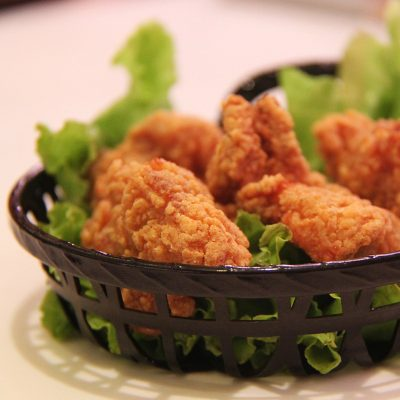Fried Chicken(2PCs) £12.00 Cras suscipit magna eget turpis tristique, at tincidunt lacus mollis.... Order

[ex_wf_grid column="5"]

### Style 1 (2 column) with PaginationIrish Cream Cake £15.00 Classic new york style cheesecake with swirls of irish cream Total: 15 if (typeof accounting === 'undefined') { (function(p,z){function q(a){return!!(""===a||a&&a.charCodeAt&&a.substr)}function m(a){return u?u(a):"[object Array]"===v.call(a)}function r(a){return"[object Object]"===v.call(a)}function s(a,b){var d,a=a||{},b=b||{};for(d in b)b.hasOwnProperty(d)&&null==a[d]&&(a[d]=b[d]);return a}function j(a,b,d){var c=[],e,h;if(!a)return c;if(w&&a.map===w)return a.map(b,d);for(e=0,h=a.length;ea?"-":"",g=parseInt(y(Math.abs(a||0),h),10)+"",l=3a?g.neg:g.zero).replace("%s",f.symbol).replace("%v",t(Math.abs(a),n(f.precision),f.thousand,f.decimal))};c.formatColumn=function(a,b,d,i,e,h){if(!a)return[];var f=s(r(b)?b:{symbol:b,precision:d,thousand:i,decimal:e,format:h},c.settings.currency),g=x(f.format),l=g.pos.indexOf("%s")a?g.neg:g.zero).replace("%s",f.symbol).replace("%v",t(Math.abs(a),n(f.precision),f.thousand,f.decimal));if(a.length>k)k=a.length;return a});return j(a,function(a){return q(a)&&a.length 1 ? '.' + x : ''; var rgx = /(\d+)(\d{3})/; while (rgx.test(x1)) { x1 = x1.replace(rgx, '\$1' + ',' + '\$2'); } return x1 + x2; } var currency = currency = ' £'; function priceformat() { //if(jQuery('.ex_modal.exfd-modal-active').length){ return;} var product_total =''; if(\$('form.variations_form').length){ if(\$('form > .single_variation_wrap .single_variation .price ins .amount').length){ product_total = jQuery('form > .single_variation_wrap .single_variation .price ins .amount').text(); } if(product_total==''){ product_total = jQuery('form > .single_variation_wrap .single_variation .price .amount').text(); } if(!\$('form > .single_variation_wrap .single_variation .price').length){ product_total = '15'; } }else{ product_total = '15'; } if(!\$.isNumeric(product_total)){ product_total = product_total.replace( currency, '' ); product_total = product_total.replace( /\,/g, '' ); product_total = product_total.replace( '.', '.' ); product_total = product_total.replace(/[^0-9\.]/g, '' ); } var _t_price = product_total; var \$qty = 1; if(\$('.quantity .qty').length){ if(jQuery('.ex_modal.exfd-modal-active').length){ \$qty = \$('.ex_modal.exfd-modal-active .quantity .qty').val(); }else{ \$qty = \$('.quantity .qty').val(); } if(jQuery.isNumeric( \$qty )){ product_total = product_total*\$qty; } } // Custom option \$('.exwo-product-options .exrow-group:not(.exwf-offrq)').each(function(){ var \$this_sl = \$(this); if(\$this_sl.hasClass('ex-radio') || \$this_sl.hasClass('ex-checkbox')){ \$this_sl.find('.ex-options').each(function(){ var \$this_op = \$(this); if(\$this_op.is(":checked")){ var \$price_op = \$this_op.data('price'); if(\$.isNumeric(\$price_op)){ if(\$this_op.data('type')=='fixed'){ product_total = product_total + \$price_op*1; }else{ product_total = product_total + (\$price_op*\$qty); } } } }); }else if(\$this_sl.hasClass('ex-select')){ \$this_sl.find('.ex-options option').each(function(){ var \$this_op = \$(this); if(\$this_op.is(":selected")){ var \$price_op = \$this_op.data('price'); if(\$.isNumeric(\$price_op)){ if(\$this_op.data('type')=='fixed'){ product_total = product_total + \$price_op*1; }else{ product_total = product_total + (\$price_op*\$qty); } } } }); }else{ var \$this_op = \$this_sl.find('.ex-options'); var \$price_op = \$this_op.data('price'); if(\$this_sl.hasClass('ex-quantity')){ \$price_op = \$price_op*\$this_sl.find('input.ex-options').val(); } if(\$this_op.val() != '' && \$.isNumeric(\$price_op)){ if(\$this_op.data('type')=='fixed'){ product_total = product_total + \$price_op; }else{ product_total = product_total + (\$price_op*\$qty); } } } }); // support product addon if(\$('#product-addons-total').length){ var addon_pr = 0; addon_pr = jQuery('#product-addons-total .price .amount').text(); if(addon_pr !=''){ addon_pr = addon_pr.replace( currency, '' ); addon_pr = addon_pr.replace( /\,/g, '' ); addon_pr = addon_pr.replace( '.', '.' ); addon_pr = addon_pr.replace(/[^0-9\.]/g, '' ); if(adult < 1){ _t_price = 0; } product_total = product_total + (adult*(addon_pr - _t_price)); \$(".wc-pao-addon-field.wc-pao-addon-checkbox").each(function(){ if(\$(this).data('price-type') =='flat_fee' && \$(this).is(':checked')){ product_total = product_total - (\$(this).data('price') * (adult -1)) } }); } } \$total_cr = accounting.formatMoney( product_total,{ symbol : currency, decimal : '.', thousand : ',', precision : '2', format : '%s%v' }); jQuery('#exlive-total .price').html( \$total_cr); } if(\$('#product-addons-total').length){ \$("body").on('DOMSubtreeModified', "#product-addons-total", function() { priceformat(); }); } jQuery('body').on('keyup mouseup change paste', '.quantity .qty', function(){ priceformat();}); jQuery('body').on('change','.variations select',function(){ priceformat(); }); jQuery('body').on('click', '#exadd_ticket', function(e) { priceformat(); }); jQuery('body').on('click', '#exminus_ticket', function(e) { priceformat(); }); jQuery('body').on('change keyup mouseup change paste', '.ex-options', function(e) { priceformat(); }); priceformat(); setTimeout(function(){ priceformat(); }, 200); }); Irish Cream Cake quantity Add to cartGarden salad £30.00 Aenean lorem augue, volutpat vitae pulvinar at, tristique vel lectus.... OrderBeef burger £8.00 – £11.00 Nulla egestas mi convallis fermentum dictum. Cras suscipit magna eget... OrderBarbecue burger £5.00 £4.00 Donec tortor erat, porta ut tellus vitae, semper faucibus quam.... Order

[ex_wf_grid count="6" posts_per_page="4"]

### Style 1 (2 column) with PaginationIrish Cream Cake £15.00 Classic new york style cheesecake with swirls of irish cream Total: 15 if (typeof accounting === 'undefined') { (function(p,z){function q(a){return!!(""===a||a&&a.charCodeAt&&a.substr)}function m(a){return u?u(a):"[object Array]"===v.call(a)}function r(a){return"[object Object]"===v.call(a)}function s(a,b){var d,a=a||{},b=b||{};for(d in b)b.hasOwnProperty(d)&&null==a[d]&&(a[d]=b[d]);return a}function j(a,b,d){var c=[],e,h;if(!a)return c;if(w&&a.map===w)return a.map(b,d);for(e=0,h=a.length;ea?"-":"",g=parseInt(y(Math.abs(a||0),h),10)+"",l=3a?g.neg:g.zero).replace("%s",f.symbol).replace("%v",t(Math.abs(a),n(f.precision),f.thousand,f.decimal))};c.formatColumn=function(a,b,d,i,e,h){if(!a)return[];var f=s(r(b)?b:{symbol:b,precision:d,thousand:i,decimal:e,format:h},c.settings.currency),g=x(f.format),l=g.pos.indexOf("%s")a?g.neg:g.zero).replace("%s",f.symbol).replace("%v",t(Math.abs(a),n(f.precision),f.thousand,f.decimal));if(a.length>k)k=a.length;return a});return j(a,function(a){return q(a)&&a.length 1 ? '.' + x : ''; var rgx = /(\d+)(\d{3})/; while (rgx.test(x1)) { x1 = x1.replace(rgx, '\$1' + ',' + '\$2'); } return x1 + x2; } var currency = currency = ' £'; function priceformat() { //if(jQuery('.ex_modal.exfd-modal-active').length){ return;} var product_total =''; if(\$('form.variations_form').length){ if(\$('form > .single_variation_wrap .single_variation .price ins .amount').length){ product_total = jQuery('form > .single_variation_wrap .single_variation .price ins .amount').text(); } if(product_total==''){ product_total = jQuery('form > .single_variation_wrap .single_variation .price .amount').text(); } if(!\$('form > .single_variation_wrap .single_variation .price').length){ product_total = '15'; } }else{ product_total = '15'; } if(!\$.isNumeric(product_total)){ product_total = product_total.replace( currency, '' ); product_total = product_total.replace( /\,/g, '' ); product_total = product_total.replace( '.', '.' ); product_total = product_total.replace(/[^0-9\.]/g, '' ); } var _t_price = product_total; var \$qty = 1; if(\$('.quantity .qty').length){ if(jQuery('.ex_modal.exfd-modal-active').length){ \$qty = \$('.ex_modal.exfd-modal-active .quantity .qty').val(); }else{ \$qty = \$('.quantity .qty').val(); } if(jQuery.isNumeric( \$qty )){ product_total = product_total*\$qty; } } // Custom option \$('.exwo-product-options .exrow-group:not(.exwf-offrq)').each(function(){ var \$this_sl = \$(this); if(\$this_sl.hasClass('ex-radio') || \$this_sl.hasClass('ex-checkbox')){ \$this_sl.find('.ex-options').each(function(){ var \$this_op = \$(this); if(\$this_op.is(":checked")){ var \$price_op = \$this_op.data('price'); if(\$.isNumeric(\$price_op)){ if(\$this_op.data('type')=='fixed'){ product_total = product_total + \$price_op*1; }else{ product_total = product_total + (\$price_op*\$qty); } } } }); }else if(\$this_sl.hasClass('ex-select')){ \$this_sl.find('.ex-options option').each(function(){ var \$this_op = \$(this); if(\$this_op.is(":selected")){ var \$price_op = \$this_op.data('price'); if(\$.isNumeric(\$price_op)){ if(\$this_op.data('type')=='fixed'){ product_total = product_total + \$price_op*1; }else{ product_total = product_total + (\$price_op*\$qty); } } } }); }else{ var \$this_op = \$this_sl.find('.ex-options'); var \$price_op = \$this_op.data('price'); if(\$this_sl.hasClass('ex-quantity')){ \$price_op = \$price_op*\$this_sl.find('input.ex-options').val(); } if(\$this_op.val() != '' && \$.isNumeric(\$price_op)){ if(\$this_op.data('type')=='fixed'){ product_total = product_total + \$price_op; }else{ product_total = product_total + (\$price_op*\$qty); } } } }); // support product addon if(\$('#product-addons-total').length){ var addon_pr = 0; addon_pr = jQuery('#product-addons-total .price .amount').text(); if(addon_pr !=''){ addon_pr = addon_pr.replace( currency, '' ); addon_pr = addon_pr.replace( /\,/g, '' ); addon_pr = addon_pr.replace( '.', '.' ); addon_pr = addon_pr.replace(/[^0-9\.]/g, '' ); if(adult < 1){ _t_price = 0; } product_total = product_total + (adult*(addon_pr - _t_price)); \$(".wc-pao-addon-field.wc-pao-addon-checkbox").each(function(){ if(\$(this).data('price-type') =='flat_fee' && \$(this).is(':checked')){ product_total = product_total - (\$(this).data('price') * (adult -1)) } }); } } \$total_cr = accounting.formatMoney( product_total,{ symbol : currency, decimal : '.', thousand : ',', precision : '2', format : '%s%v' }); jQuery('#exlive-total .price').html( \$total_cr); } if(\$('#product-addons-total').length){ \$("body").on('DOMSubtreeModified', "#product-addons-total", function() { priceformat(); }); } jQuery('body').on('keyup mouseup change paste', '.quantity .qty', function(){ priceformat();}); jQuery('body').on('change','.variations select',function(){ priceformat(); }); jQuery('body').on('click', '#exadd_ticket', function(e) { priceformat(); }); jQuery('body').on('click', '#exminus_ticket', function(e) { priceformat(); }); jQuery('body').on('change keyup mouseup change paste', '.ex-options', function(e) { priceformat(); }); priceformat(); setTimeout(function(){ priceformat(); }, 200); }); Irish Cream Cake quantity Add to cartGarden salad £30.00 Aenean lorem augue, volutpat vitae pulvinar at, tristique vel lectus.... OrderBeef burger £8.00 – £11.00 Nulla egestas mi convallis fermentum dictum. Cras suscipit magna eget... OrderBarbecue burger £5.00 £4.00 Donec tortor erat, porta ut tellus vitae, semper faucibus quam.... Order

[ex_wf_grid count="6" posts_per_page="4"]